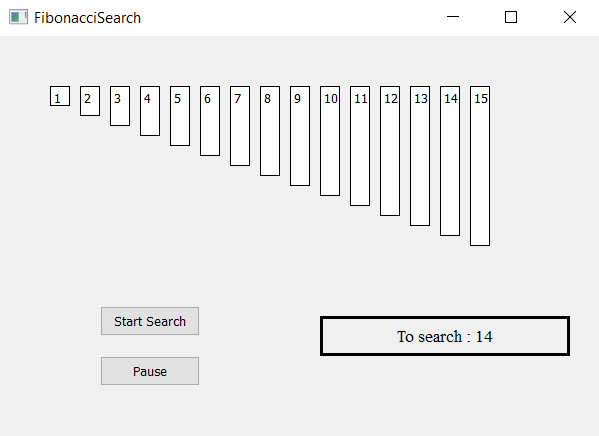Related Articles

# Fibonacci Search Visualizer using PyQt5

• Last Updated : 02 Jun, 2021

In this article we will see how we can make a PyQt5 application which will visualize the exponential search algorithm.

Fibonacci search technique is a method of searching a sorted array using a divide and conquer algorithm that narrows down possible locations with the aid of Fibonacci numbers. Compared to binary search where the sorted array is divided into two equal-sized parts, one of which is examined further, Fibonacci search divides the array into two parts that have sizes that are consecutive Fibonacci numbers.GUI implementation steps :
1. Create a list of label according to the given list of numbers
2. Set their text, border, color and geometry with respective gap from each other
3. Each label height should be proportional to the value of each number
4. Create a start and pause push button to start the searching and pause the searching
5. Create a result label to show the searching status
Back end implementation steps :
1. Create label list corresponding to the given numbers
2. Create variable for the index used by Fibonacci search and flag for searching and flag for searching    minimum Fibonacci number and for searching value after getting the Fibonacci number
3. Add action to the push button their action should change the flag status i.e start action should make    flag true and pause action should make flag false
4. Create timer object which calls a method after every specific time
5. Inside the timer method check for the flag is flag is true begin the search of Fibonacci number
6. After finding the fibonacci number find the given number within the range
7. Show the result

Below is the implementation

## Python3

 `# importing libraries``from` `PyQt5.QtWidgets ``import` `*``from` `PyQt5 ``import` `QtCore, QtGui``from` `PyQt5.QtGui ``import` `*``from` `PyQt5.QtCore ``import` `*``import` `sys`  `class` `Window(QMainWindow):``    ``# list of numbers``    ``number ``=` `[``1``, ``2``, ``3``, ``4``, ``5``, ``6``, ``7``, ``8``, ``9``, ``10``, ``11``, ``12``, ``13``, ``14``, ``15``]` `    ``# desired list``    ``desired ``=` `14` `    ``def` `__init__(``self``):``        ``super``().__init__()` `        ``# setting title``        ``self``.setWindowTitle(``"Fibonacci Search"``)` `        ``# setting geometry``        ``self``.setGeometry(``100``, ``100``, ``600``, ``400``)` `        ``# calling method``        ``self``.UiComponents()` `        ``# showing all the widgets``        ``self``.show()` `    ``# method for widgets``    ``def` `UiComponents(``self``):` `        ``# start flag``        ``self``.start ``=` `False` `        ``# divide flag``        ``self``.divide ``=` `False``        ``self``.fib_search ``=` `True` `        ``# list to hold labels``        ``self``.label_list ``=` `[]` `        ``# fibonacci numbers``        ``self``.fib1 ``=` `1``        ``self``.fib2 ``=` `0``        ``self``.fib ``=` `self``.fib1 ``+` `self``.fib2` `        ``self``.offset ``=` `-``1` `        ``# local counter``        ``c ``=` `0` `        ``# iterating list of numbers``        ``for` `i ``in` `self``.number:``            ``# creating label for each number``            ``label ``=` `QLabel(``str``(i), ``self``)` `            ``# adding background color and border``            ``label.setStyleSheet("border : ``1px` `solid black;``                                 ``background : white;")` `            ``# aligning the text``            ``label.setAlignment(Qt.AlignTop)` `            ``# setting geometry using local counter``            ``# first parameter is distance from left``            ``# and second is distance from top``            ``# third is width and forth is height``            ``label.setGeometry(``50` `+` `c ``*` `30``, ``50``, ``20``, i ``*` `10` `+` `10``)` `            ``# adding label to the label list``            ``self``.label_list.append(label)` `            ``# incrementing local counter``            ``c ``=` `c ``+` `1` `        ``# creating push button to start the search``        ``self``.search_button ``=` `QPushButton(``"Start Search"``, ``self``)` `        ``# setting geometry of the button``        ``self``.search_button.setGeometry(``100``, ``270``, ``100``, ``30``)` `        ``# adding action to the search button``        ``self``.search_button.clicked.connect(``self``.search_action)` `        ``# creating push button to pause the search``        ``pause_button ``=` `QPushButton(``"Pause"``, ``self``)` `        ``# setting geometry of the button``        ``pause_button.setGeometry(``100``, ``320``, ``100``, ``30``)` `        ``# adding action to the search button``        ``pause_button.clicked.connect(``self``.pause_action)` `        ``# creating label to show the result``        ``self``.result ``=` `QLabel(``"To search : "` `+` `str``(``self``.desired), ``self``)` `        ``# setting geometry``        ``self``.result.setGeometry(``320``, ``280``, ``250``, ``40``)` `        ``# setting style sheet``        ``self``.result.setStyleSheet(``"border : 3px solid black;"``)` `        ``# adding font``        ``self``.result.setFont(QFont(``'Times'``, ``10``))` `        ``# setting alignment``        ``self``.result.setAlignment(Qt.AlignCenter)` `        ``# creating a timer object``        ``timer ``=` `QTimer(``self``)` `        ``# adding action to timer``        ``timer.timeout.connect(``self``.showTime)` `        ``# update the timer every 300 millisecond``        ``timer.start(``300``)` `    ``# method called by timer``    ``def` `showTime(``self``):` `        ``# checking if flag is true``        ``if` `self``.start:` `            ``# search fibonacci number``            ``if` `self``.fib_search:` `                ``# searching for the Fibonacci number greater``                ``# then the desired number``                ``if` `self``.fib < ``len``(``self``.number):` `                    ``self``.fib2 ``=` `self``.fib1``                    ``self``.fib1 ``=` `self``.fib``                    ``self``.fib ``=` `self``.fib2 ``+` `self``.fib1``                    ``self``.result.setText(``"Searching Fibonacci number >="` `+``                                                        ``str``(``self``.desired))`  `                ``# start divide search``                ``else``:``                    ``self``.result.setText(``"Fibonacci found, searching number"``)``                    ``self``.fib_search ``=` `False``                    ``self``.divide ``=` `True`  `            ``# start divide search``            ``if` `self``.divide:` `                ``if` `self``.fib <``=` `1``:``                    ``self``.result.setText(``"Not found"``)``                    ``self``.start ``=` `False``                    ``return` `                ``i ``=` `min``(``self``.offset ``+` `self``.fib2, ``len``(``self``.number) ``-` `1``)` `                ``self``.label_list[i].setStyleSheet(``"border : 1px solid black;"``                                                 ``"background-color : grey"``)` `                ``# If desired is greater than the value at``                ``# index fib2, cut the subarray array``                ``# from offset to i``                ``if` `(``self``.number[i] < ``self``.desired):``                    ``self``.fib ``=` `self``.fib1``                    ``self``.fib1 ``=` `self``.fib2``                    ``self``.fib2 ``=` `self``.fib ``-` `self``.fib1``                    ``self``.offset ``=` `i` `                ``# If desired is greater than the value at``                ``# index fib2, cut the subarray``                ``# after i + 1``                ``elif` `(``self``.number[i] > ``self``.desired):``                    ``self``.fib ``=` `self``.fib2``                    ``self``.fib1 ``=` `self``.fib1 ``-` `self``.fib2``                    ``self``.fib2 ``=` `self``.fib ``-` `self``.fib1` `                ``# element found. show result and stop search``                ``else``:``                    ``self``.result.setText(``"Found at : "` `+` `str``(i))``                    ``self``.label_list[i].setStyleSheet(``                                 ``"border : 2px solid green;"``                                 ``"background-color : lightgreen;"``)``                    ``self``.start ``=` `False`    `    ``# method called by search button``    ``def` `search_action(``self``):` `        ``# making flag true``        ``self``.start ``=` `True` `        ``# showing text in result label``        ``self``.result.setText(``"Started searching..."``)` `    ``# method called by pause button``    ``def` `pause_action(``self``):` `        ``# making flag false``        ``self``.start ``=` `False` `        ``# showing text in result label``        ``self``.result.setText(``"Paused"``)`  `# create pyqt5 app``App ``=` `QApplication(sys.argv)` `# create the instance of our Window``window ``=` `Window()` `# start the app``sys.exit(App.``exec``())`

Output :

Attention geek! Strengthen your foundations with the Python Programming Foundation Course and learn the basics.

To begin with, your interview preparations Enhance your Data Structures concepts with the Python DS Course. And to begin with your Machine Learning Journey, join the Machine Learning – Basic Level Course

My Personal Notes arrow_drop_up